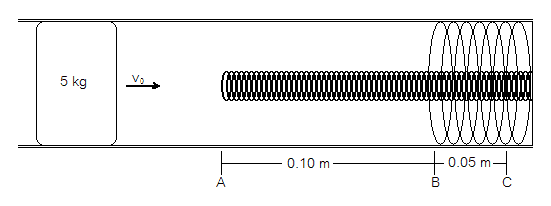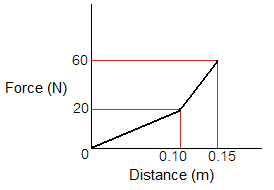# Calculating the decrease in kinetic energy of an object as it moves against a spring

shandsy

## Homework Statement

A 5-kg object initially slides with speed V0 in a hollow frictionless pipe. The end of the pipe contains two springs, one nested inside the other, as shown above. The object makes contact with the inner spring at point A, moves 0.1 meter to make contact with the outer spring at point B, and then moves an additional 0.05 meter before coming to rest at point C. The graph shows the magnitude of the force exerted on the object by the springs as a function of the object's distance from point A.
a. Calculate the spring constant for the inner spring.​
b. Calculate the decrease in kinetic energy of the object as it moves from point A to point B.​
c. Calculate the additional decrease in kinetic energy of the object as it moves from point B to point C.​
d. Calculate the initial speed V0 of the object.​
e. Calculate the spring constant of the outer spring.​

m = 5 kg
ΔxA-B = 0.10 m
ΔxB-C = 0.05 m
Vat C = 0 m/s

(Here are the diagram and the graph. Sorry if they're less than beautiful, I just quickly recreated them on MS Paint)## Homework Equations

F=kx
Uelastic=(1/2)kx2
Kinematic Equations

## The Attempt at a Solution

According to the graph, F=20 N when the object is at B, and it's displacement at B (relative to the point at which it first made contact with the inner spring) is 0.10 m. So:
F = kx
20N = k(0.10m)
k = 200 N/m

As for part b, I don't really know where to start. Would I calculate Uelastic to find the decrease in kinetic energy? I have no idea how to do c, d, or e, either...

## Answers and Replies

Hypersphere

Use energy conservation. The loss in kinetic energy corresponds to an increase in the potential energy due to the spring.

azizlwl

According to the graph, F=20 N when the object is at B, and it's displacement at B (relative to the point at which it first made contact with the inner spring) is 0.10 m. So:
F = kx
20N = k(0.10m)
k = 200 N/m
----------
F is not constant.
You can find work done on the spring, Fx, as an area under the graph.
The work done on the spring is stored as potential energy, 1/2 kx2